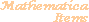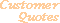Home Older Mathematica Items

Scientific Arts

# Linear and Nonlinear Least Squares Fitting to Data

## Linear Least Squares Fits: Specific Heats in H20

This data comes from the NIST WebBook:

 E.W. Lemmon, M.O. McLinden and D.G. Friend, "Thermophysical Properties of Fluid Systems"  in NIST Chemistry WebBook, NIST Standard Reference Database Number 69, Eds. W.G. Mallard and P.J. Linstrom, November 1998, National Institute of Standards and Technology, Gaithersburg MD, 20899 (http://webbook.nist.gov).

We have placed the information in the file h2odata.dat. First read it into the variable H2OData. (If the file h2odata.dat is not placed in a directory that is contained in Mathematica's \$Path variable, you can make it available by executing the functionwhere path is the path to the needed directory.)

``````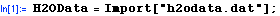``````

The first line in h2odata.dat contains information on the data that is contained in each column (there are 11 columns of data):

``````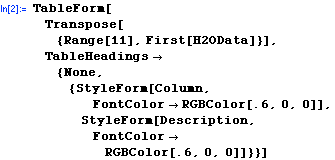``````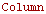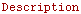1 Temperature(K) 2 Pressure(atm) 3 Density(mol/l) 4 Volume(l/mol) 5 InternalEnergy(kJ/mol) 6 Enthalpy(kJ/mol) 7 Entropy(J/mol*K) 8 Cv(J/mol*K) 9 Cp(J/mol*K) 10 SoundSpeed(m/s) 11 Phase

Now remove the first (header) line to redefine H2OData.

``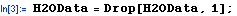``

Create a variable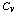by extracting the specific heat at constant volume versus temperature (this command extracts columns 1 and 8 from H2OData to create a list of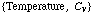data points).

``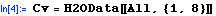``
```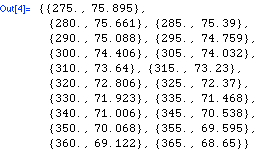```

Create another variable,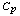, by extracting the specific heat at constant pressure versus temperature.

``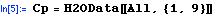``
```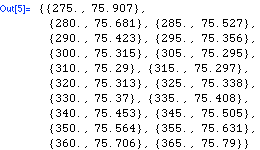```

Now make plots of both data sets.

``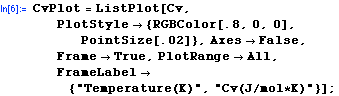``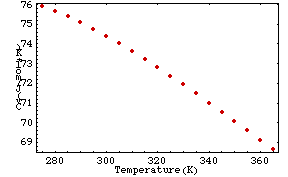``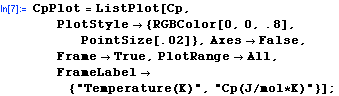``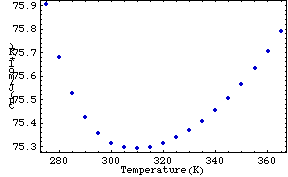Here they are together in one plot.

``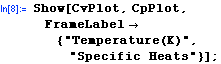``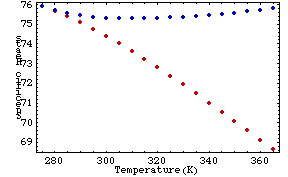Now use Fit to find a least squares fit of thedata to a cubic polynomial of the form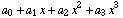.  We also compute a quadratic fit for comparison.

Here are the fits.

``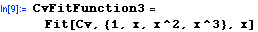``
```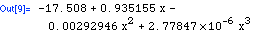```
````
```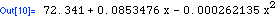```

Now plot all three fits together. The plot is suppressed through the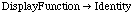option, but we give it the name fitPlotCv so that we can use it below.

``````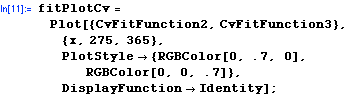``````

Now show these three plots along with the data.

``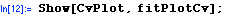``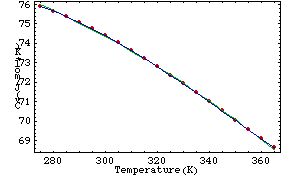Now let's do the same procedure with thedata.

``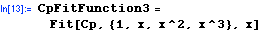``
```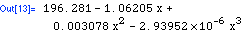```
``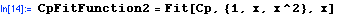``
```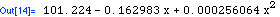```
``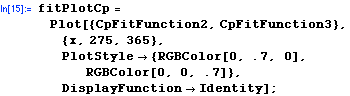``
``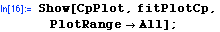``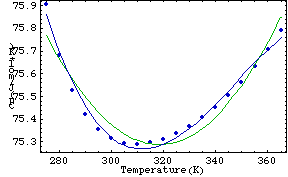The standard add-on package Statistics`LinearRegression` extends the functionality of Fit by allowing access to a great deal of detail about the statistical properties of the resulting fit.

``````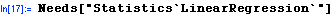``````

Here is an example.

``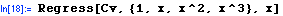``
```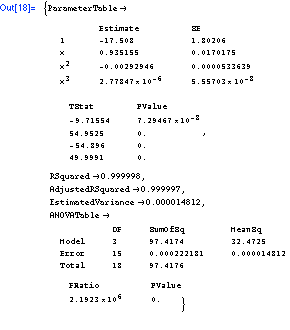```

Here is a list of the estimated variances for linear, quadratic, and cubic fits. First thedata:

``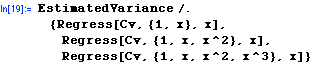``
`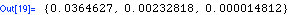`

Now the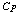data:

``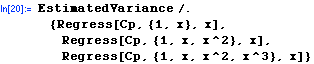``
```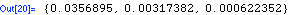```

## Nonlinear Fits: Fitting to a Gaussian Function

### Generate Some Artificial Data

We will first create a data set based on normally distributed random numbers. The standard add-on package Statistics`NormalDistribution` extends the built in function Random[] so that it can generate normally distributed random numbers. The standard add-on package Statistics`ContinuousDistributions` generalizes Random[] to a large variety of other probability distributions (if you load Statistics`ContinuousDistributions`, then Statistics`NormalDistribution` is automatically loaded as well).

First load the package (we also load the standard add-on package Statistics`DataManipulation` to enable the BinCounts function that allows us to easily bin the data that we will generate).

``````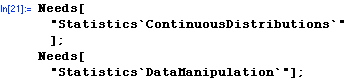``````

Here is the symbolic expression for the normal probability density function.

``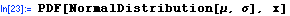``
```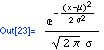```

Now generate 1000 random normally distributed numbers with a mean of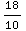and a standard deviation of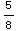.

``````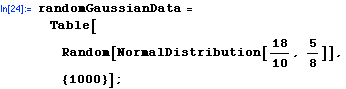``````

Now bin the data using BinCounts. The result is normalized to a total probability of one, and the amount in each bin is associated with the value of the bin's center point.

``````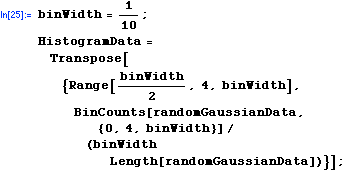``````

### Analysis of Gaussian Data

Here is the plot of the data that we generated above along with the normal curve that it was based upon.

``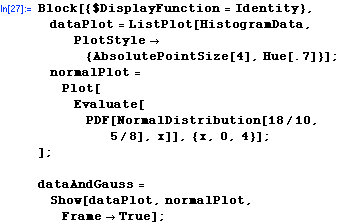``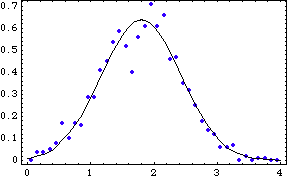If we did not know that this data had come from a normal distribution but nonetheless wished to fit it to a parameterized Gaussian function, we could make use of the nonlinear fitting functions provided by the standard add-on package Statistics`NonlinearFit` (which, among other methods, implements the Levenberg-Marquardt method). First we load the package.

``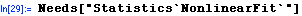``

Now we use NonlinearFit, guessing starting values for the parameters based on the graph above.

``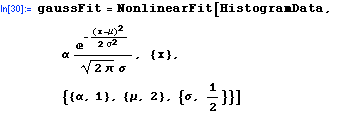``
```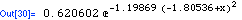```

Here is the fit function graphically compared to the data and the original Gaussian function.

``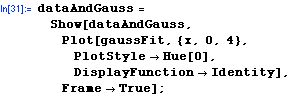``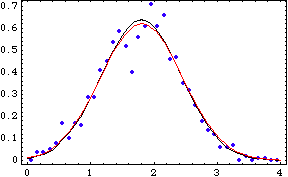While NonlinearFit returns the parameterized fitting function, NonlinearRegress returns a list of rules that specify, in addition to the fitting parameters, a variety of statistical quantities associated with the fit. (There is much more information that can be returned from NonlinearRegress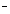through the RegressionReport optionin addition to the set shown here: see the documentation for Statistics`NonlinearFit` for more detail.)

``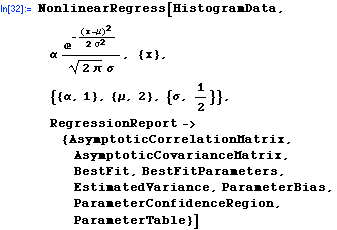``
`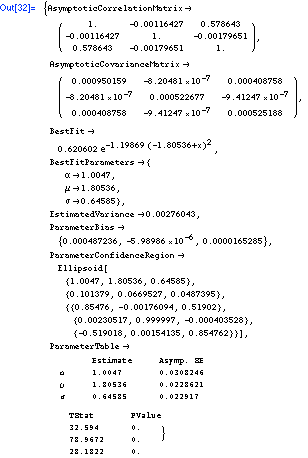`
 For further information on our services send email to info@scientificarts.com . Contents of this web site Copyright © 1999-2011, Scientific Arts, LLC.
 s